Latest Banking jobs   »

# Quantitative Aptitude Quiz For Bank Foundation 2023 11th January

Direction (1-5): The following questions are accompanied by two statements (I) and statement (II). You have to determine which statements(s) is/are sufficient/necessary to answer the questions.
(a) Neither statement (I) nor statement (II) by itself is sufficient to answer the question.
(b) Statement (II) alone is sufficient to answer the question but statement (I) alone is not sufficient to answer the question.
(c) Either statement (I) or statement (II) by itself is sufficient to answer the question.
(d) Both the statements taken together are necessary to answer the questions, but neither of the statements alone is sufficient to answer the question.
(e) Statement (I) alone is sufficient to answer the question but statement (II) alone is not sufficient to answer the questions

Q1. If C’s height is 50 cm, then find the sum of height of A, B and C together.
Statement I: The sum of the heights of A and C is equal to 160 cm.
Statement II: The height of B is equal to 3 times the height of C.

Q2. The cost price of an article is Rs.100. What is the profit earned by the shopkeeper on selling the article?
Statement I: The marked price of the article was 140% of the cost price.
Statement II: A discount of 20% on the marked price was given on the article.

Q3. If two taps P and Q are open simultaneously, then find how long would it take to fill 50% capacity of the tank?
Statement I: P can fill the empty tank in 12 hours.
Statement II: Q can empty 50% tank in 10 hours.

Q4. What is the sum of x and y?
Statement I : 15x + 4y = 108
Statement II : y = 2 + 15m

Q5. A sum of Rs.180 was distributed among A, B and C. Who among the three got the highest share?
Statement I : The share of A was one fifth of the share of B and C together.
Statement II : The respective ratio of share of C, B and A is 3:2:1.

Directions (6–10): In the given questions, two quantities are given, one as ‘Quantity I’ and another as ‘Quantity II’. You have to determine relationship between two quantities and choose the appropriate option.

Q6. Quantity I: 2x²– 23x + 56 = 0
Quantity II: II. 4x²– 19x + 12 = 0
(a) Quantity I > Quantity II
(b) Quantity I < Quantity II
(c) Quantity I ≥ Quantity II
(d) Quantity I ≤ Quantity II
(e) Quantity I = Quantity II or no relation

Q7. Quantity I: P starts business by investing Rs.4500 and after 7 months, Q joins the business. After a year, the profit divides between P and Q in the ratio of 3 : 5 respectively. Find the investment of Q.
Quantity II: Rs.16000
(a) Quantity I > Quantity II
(b) Quantity I < Quantity II
(c) Quantity I ≥ Quantity II
(d) Quantity I ≤ Quantity II
(e) Quantity I = Quantity II or no relation

Q8. Quantity I: Rs.840
Quantity II: A sum of Rs.800 amounts to Rs.920 in two years at simple interest. If the interest rate is increase by 2.5%, then find the amount received on new interest rate after two years.
(a) Quantity I > Quantity II
(b) Quantity I < Quantity II
(c) Quantity I ≥ Quantity II
(d) Quantity I ≤ Quantity II
(e) Quantity I = Quantity II or no relation

Q9. Quantity I: The average age of 21 people is 64 years. If the age of a new person is added the new average age becomes 64.5 years, then find the age of new person.
Quantity II: There are 14 people in a family and the average age of all the family members is 30 years. A new baby born in a family, after 4 years what will be the average age of the all-family member.
(a) Quantity I > Quantity II
(b) Quantity I < Quantity II
(c) Quantity I ≥ Quantity II
(d) Quantity I ≤ Quantity II
(e) Quantity I = Quantity II or no relation

Q10. Quantity I: (625)1/2 y²– 495 = √16900
Quantity II: x=30
(a) Quantity I > Quantity II
(b) Quantity I < Quantity II
(c) Quantity I ≥ Quantity II
(d) Quantity I ≤ Quantity II
(e) Quantity I = Quantity II or no relation

Directions (11–15): In the given questions, two quantities are given, one as ‘Quantity I’ and another as ‘Quantity II’. You have to determine relationship between two quantities and choose the appropriate option

Q11. Quantity I. In the annual function of a school 30% student left at 8pm, 20% of the remaining left at 9pm and only 42210 students left at the end. Find the total number of students who were present in the annual function?
Quantity II. The population of a town is 126000. It decreases by 50% and 20% in the first year and second year respectively. What is the population of the town at the end of 2 year?
(a) Quantity I > Quantity II
(b) Quantity I < Quantity II
(c) Quantity I ≥ Quantity II
(d) Quantity I ≤ Quantity II
(e) Quantity I = Quantity II or no relation

Q12. Quantity I. The difference of two numbers is 180. If 25% of one number is 75% of the other number, then find the smallest number?
Quantity II. 90
(a) Quantity I > Quantity II
(b) Quantity I < Quantity II
(c) Quantity I ≥ Quantity II
(d) Quantity I ≤ Quantity II
(e) Quantity I = Quantity II or no relation

Q13. Quantity I. A student has to secure 40% marks to pass but he gets 178 marks and fails by 22 marks. Find the maximum marks?
Quantity II. 550
(a) Quantity I > Quantity II
(b) Quantity I < Quantity II
(c) Quantity I ≥ Quantity II
(d) Quantity I ≤ Quantity II
(e) Quantity I = Quantity II or no relation

Q14. Quantity I: x²+16x+63=0
Quantity II: x²+25x+144=0
(a) Quantity I > Quantity II
(b) Quantity I < Quantity II
(c) Quantity I ≥ Quantity II
(d) Quantity I ≤ Quantity II
(e) Quantity I = Quantity II or No relation

Q15. Quantity I: 3x²+22x+40=0
Quantity II: x²-16x-36=0
(a) Quantity I > Quantity II
(b) Quantity I < Quantity II
(c) Quantity I ≥ Quantity II
(d) Quantity I ≤ Quantity II
(e) Quantity I = Quantity II or No relation

Solutions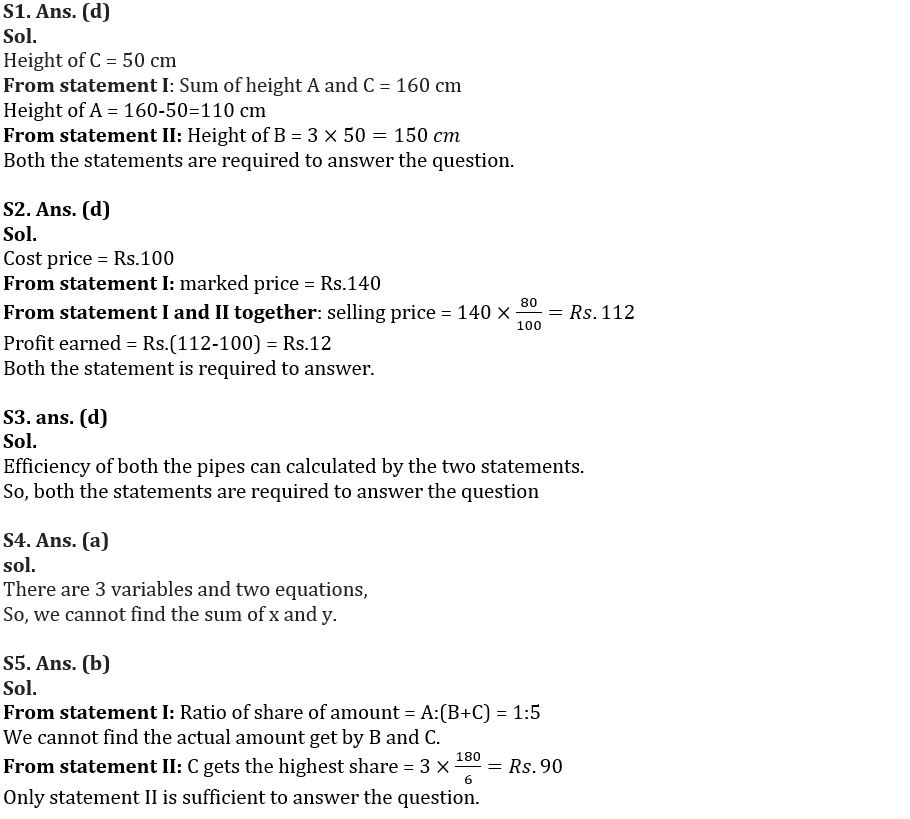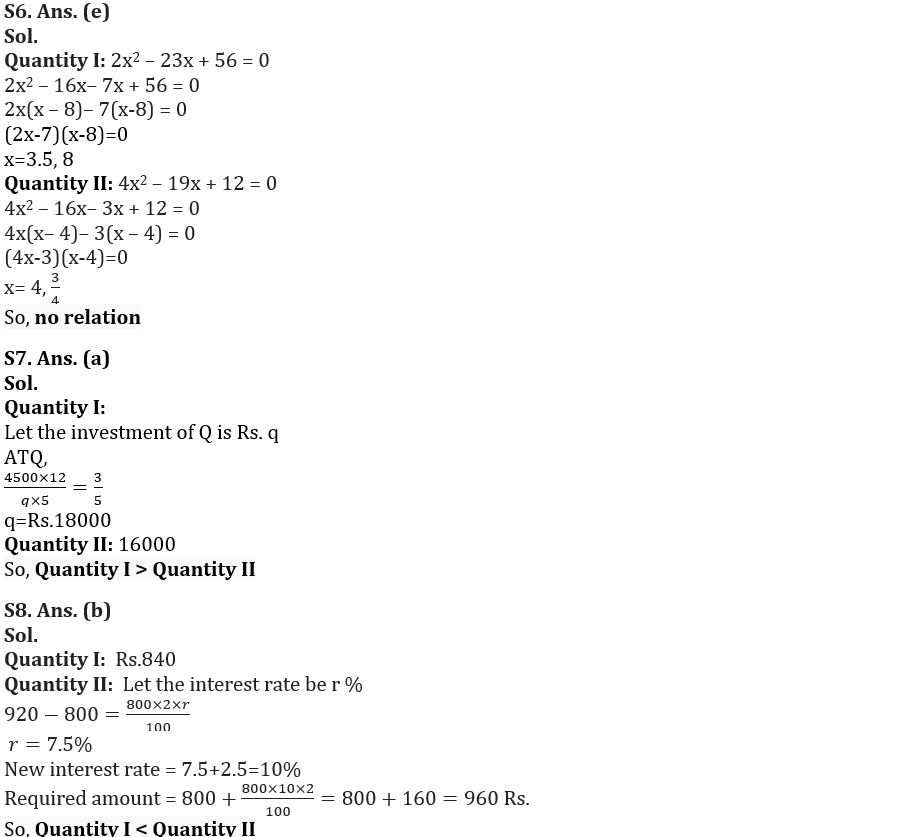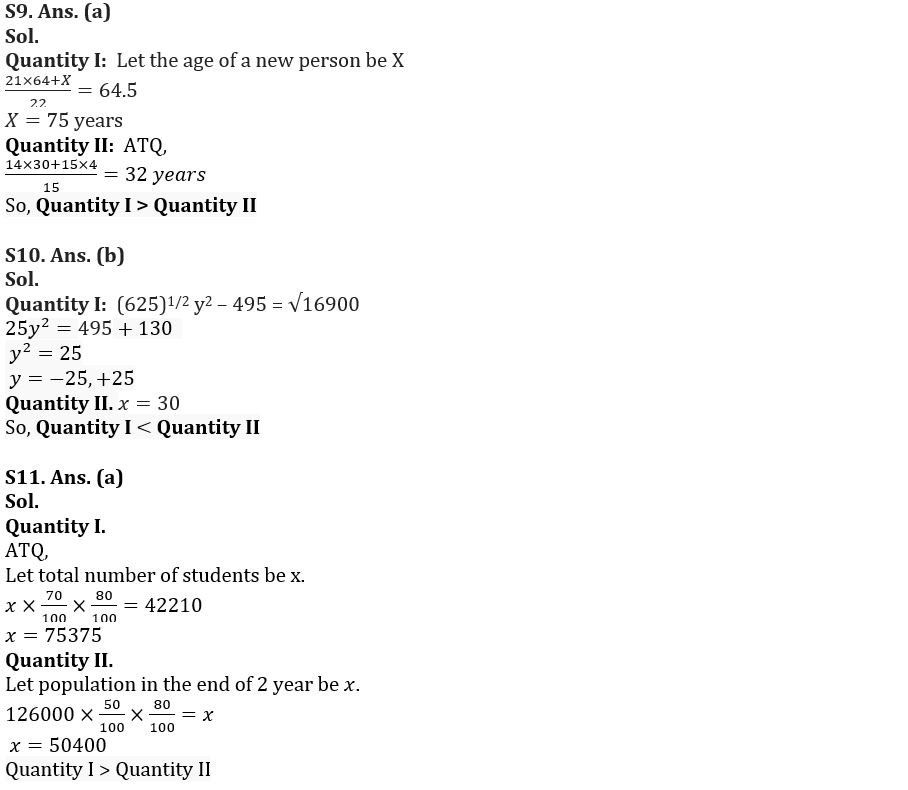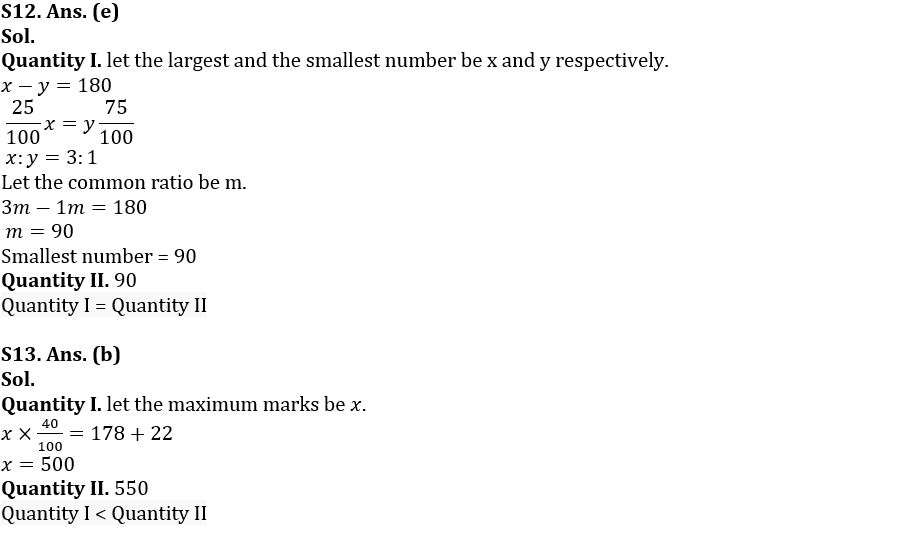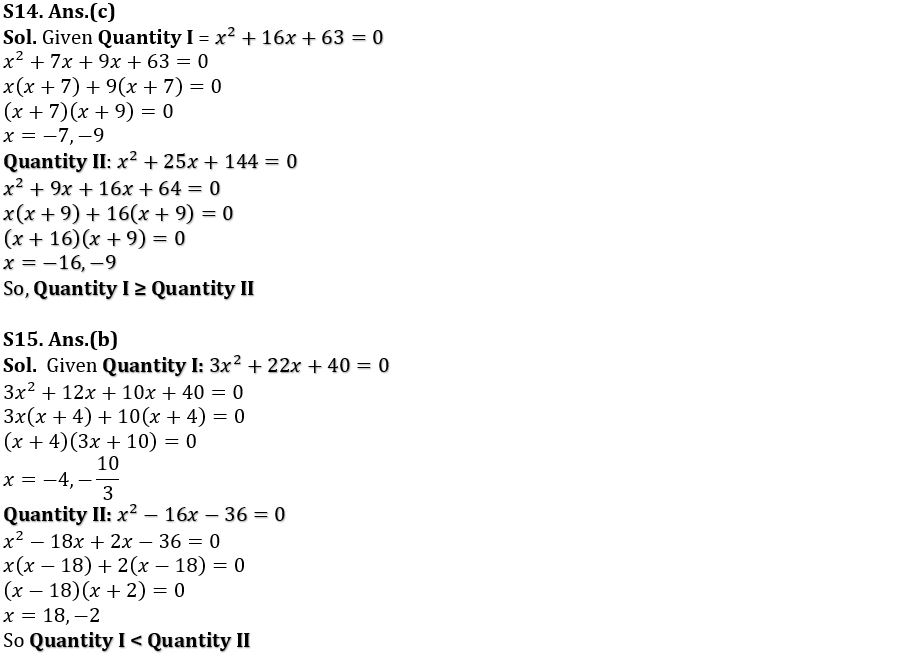## FAQs

### What is the selection process of the Bank Clerk?

The selection process of the Bank Clerk is Prelims & Mains.

#### Congratulations!Union Budget 2023-24: Free PDF